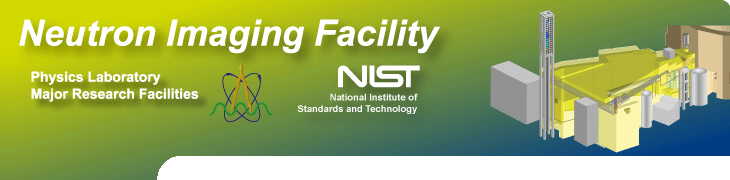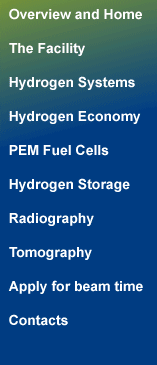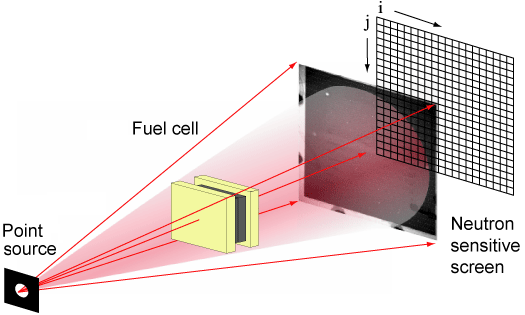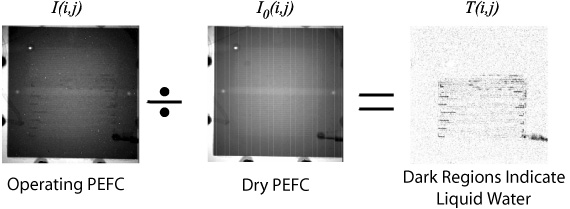Neutron radiographs are the neutron shadows of objects placed in the beam. Using a 2 dimensional neutron camera the image captured is actually a gray level image where the gray level of each pixel is proportional to the number of neutrons that made it through the object. This gray level value, or the number of measured neutrons is proportional to the amount of neutron scattering and absorbing material in the beam and is determined from the attenuation law known as Beers Law:

I(i,j ) = I0(i,j ) exp(-µ t).
In this case, µ is known as the attenuation length of the material. A large µ means that a material will strongly scatter or absorb neutrons. One can measure µ in a separate experiment (for instance by using a stepped wedge of water, or a cuvet) and thus obtain the material thickness, t, in a pixel. Since the area of a pixel is known, this thickness can be converted to either a volume or mass of liquid water. More information on the measurement of µ can be found water calibration.

The first step to measuring water is to form the transmission image. By dividing the image during operation by a reference image (either of the beam without a sample, or the sample in a dry state) one arrives at the transmission image. The transmission image allows one to quantify the amount of material in the beam and to do numerical analysis with the images. Small amounts of water in the operating fuel cell which are barely visible in the operating image are dramatically apparent in the transmission image.